Midterm 2 Preparation

# Midterm 2: Version D

Find the solution set of the system graphically.

1.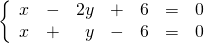For problems 2–4, find the solution set of each system by any convenient method.

1.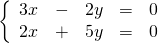2.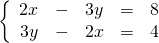3.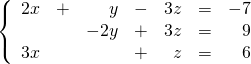Reduce the following expressions in questions 5–7.

1.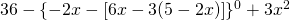2.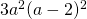3.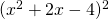Divide using long division.

1.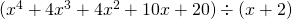For problems 9–12, factor each expression completely.

1.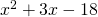2.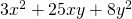3.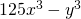4.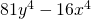Solve the following word problems.

1. The sum of the ages of a boy and a girl is 18 years. Four years ago, the girl was four times the age of the boy. Find the present age of each child.
2. A purse contains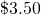made up of dimes and quarters. If there are 20 coins in all, how many dimes and how many quarters were there?
3. A 60 kg blend of two different grades of tea is sold for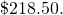If grade A sells for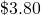per kg and grade B sells for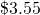per kg, how many kg of each grade were used?